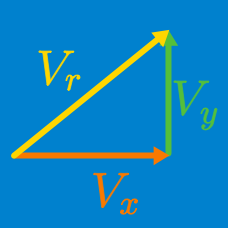Classical Mechanics

# Total displacement from velocity vectors

A rocket initially at $(0,0)$ moves with a constant velocity of $(7\text{ m/s},6\text{ m/s})$ through vacuum. After moving for $54\text{ s},$ an asteroid hits the rocket and a change of $(1\text{ m/s},0)$ is made to the rocket's velocity. What is the position vector of the rocket at $t=60\text{ s}?$

A man initially at $(0,0)$ on the coordinate plane walks with a velocity of $(3\text{ m/s},-\sqrt{5}\text{ m/s})$ for $2$ seconds. Then he walks with a velocity of $(-1\text{ m/s}, 1\text{ m/s})$ for $3$ seconds. Find the magnitude of his total displacement.

A kayaker paddles due north at 2 m/s. The river the kayaker is paddling in flows towards the east at 1 m/s. After 5 seconds of paddling, how far is the kayaker from where she started in meters?

You wish to swim across a small river to your friend on the other side. The river is 30 meters wide and you begin to swim straight across it at a speed of 1 m/s. The current in the river is 0.5 m/s and so the river carries you downriver as you swim. How far downriver in meters are you when you get to the other side?

A train is moving at a constant speed of $80\text{ km/h}.$ The train first moves in the $+x$ direction for an hour. Then it turns $60^{\circ}$ upward and moves for two hours. Find the magnitude of the train's total displacement.

×